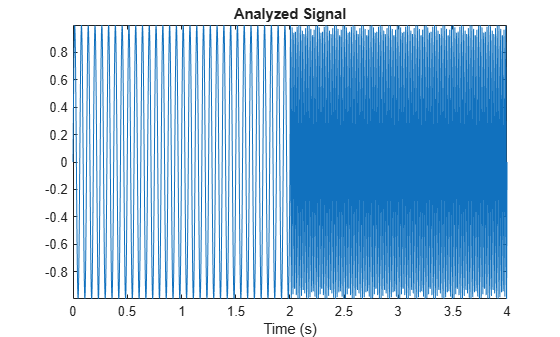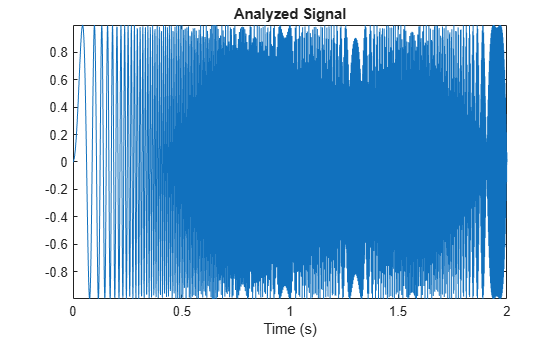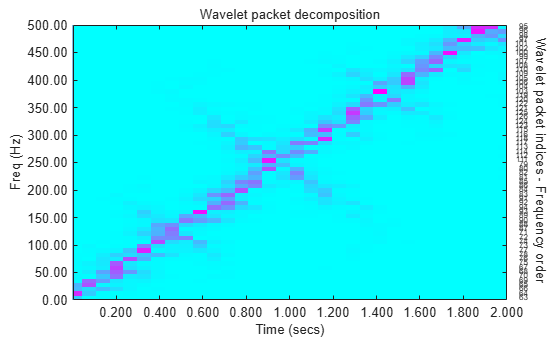# wpspectrum

Wavelet packet spectrum

## Syntax

```[SPEC,TIMES,FREQ] = wpspectrum(WPT,Fs) [...] = wpspectrum(WPT,Fs,'plot') [...,TNFO] = wpspectrum(...) ```

## Description

```[SPEC,TIMES,FREQ] = wpspectrum(WPT,Fs)``` returns a matrix of wavelet packet spectrum estimates, `SPEC`, for the binary wavelet packet tree object, `WPT`. `Fs` is the sampling frequency in Hertz. `SPEC` is a 2J-by-N matrix where J is the level of the wavelet packet transform and N is the length of the time series. `TIMES` is a 1-by-N vector of times and `FREQ` is a 1-by-2J vector of frequencies.

`[...] = wpspectrum(WPT,Fs,'plot')` displays the wavelet packet spectrum.

`[...,TNFO] = wpspectrum(...)` returns the terminal nodes of the wavelet packet tree in frequency order.

## Input Arguments

 `WPT` `WPT` is a binary wavelet packet tree of class `wptree`. `Fs` Sampling frequency in Hertz as a scalar of class double. Default: 1 `plot` The character vector `'plot'` displays the wavelet packet spectrum. Enter `'plot'` after `Fs` to produce a plot of the wavelet packet spectrum.

## Output Arguments

 `SPEC` Wavelet packet spectrum. `SPEC` is a 2J-by-N matrix where J is the level of the wavelet packet transform and N is the length of node 0 in the wavelet packet tree object. The frequency spacing between the rows of `SPEC` is Fs/2J+1. `TIMES` Time vector. `TIMES` is a vector of times in seconds equal in length to node 0 of the wavelet packet tree object. The time spacing between elements is 1/Fs. `FREQ` Frequency vector. `FREQ` is a vector of frequencies of length 2J where J is the level of the wavelet packet tree object. The frequency spacing in `FREQ` is Fs/2J+1. `TNFO` Terminal nodes. `TNFO` is a vector of the terminal nodes of the wavelet packet tree object in frequency order.

## Examples

collapse all

This example shows wavelet packet spectrum for signal consisting of two sinusoids with disjoint support.

Define wavelet.

```fs = 500; t = 0:1/fs:4; y = sin(32*pi*t).*(t<2) + sin(128*pi*t).*(t>=2); plot(t,y); axis tight title('Analyzed Signal');```Define wavelet packet spectrum.

```level = 6; wpt = wpdec(y,level,'sym6'); figure; [S,T,F] = wpspectrum(wpt,fs,'plot');```Create the chirp signal.

```fs = 1000; t = 0:1/fs:2; % create chirp signal y = sin(256*pi*t.^2);```

Plot the analyzed signal.

```plot(t,y); axis tight title('Analyzed Signal');```Get the wavelet packet spectrum estimates.

```level = 6; wpt = wpdec(y,level,'sym8'); figure; [S,T,F] = wpspectrum(wpt,fs,'plot');```collapse all

### Wavelet Packet Spectrum

The wavelet packet spectrum contains the absolute values of the coefficients from the frequency-ordered terminal nodes of the input binary wavelet packet tree. The terminal nodes provide the finest level of frequency resolution in the wavelet packet transform. If J denotes the level of the wavelet packet transform and Fs is the sampling frequency, the terminal nodes approximate bandpass filters of the form:

`$\left[\frac{nFs}{{2}^{J+1}},\frac{\left(n+1\right)Fs}{{2}^{J+1}}\right)\text{ }n=0,1,2,3,\dots {2}^{J}-1$`

At the terminal level of the wavelet packet tree, the transform divides the interval from 0 to the Nyquist frequency into bands of approximate width $Fs/{2}^{J+1}.$

## Algorithms

`wpspectrum` computes the wavelet packet spectrum as follows:

• Extract the wavelet packet coefficients corresponding to the terminal nodes. Take the absolute value of the coefficients.

• Order the wavelet packet coefficients by frequency ordering.

• Determine the time extent on the original time axis corresponding to each wavelet packet coefficient. Repeat each wavelet packet coefficient to fill in the time gaps between neighboring wavelet packet coefficients and create a vector equal in length to node 0 of the wavelet packet tree object.

## References

Wickerhauser, M.V. Lectures on Wavelet Packet Algorithms, Technical Report, Washington University, Department of Mathematics, 1992.

### Topics

Introduced in R2010b

## SupportGet trial now